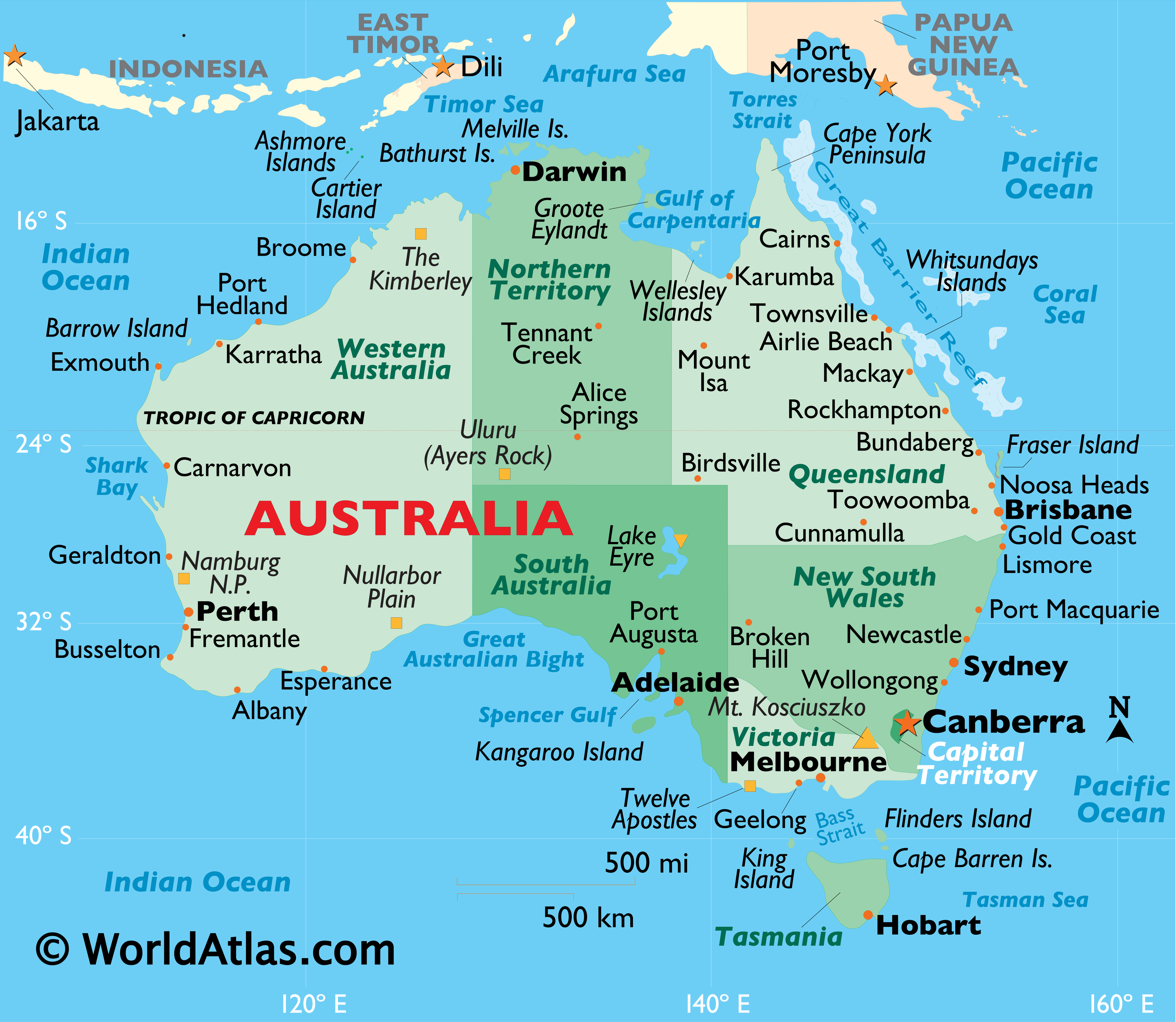####spherical

••214##### Timezone Northern and Southern Hemisphere
« on: April 26, 2019, 09:55:59 PM »
Can you please, inform what is the distance in kilometers or miles between time zones on the latitude 28.5°N (Orlando FL) and 28.5°S (Rio Grande do Sul Brazil) ?   Considering that all 24 time zones lines concentrate on the North Pole, on the flat earth design they open up like an umbrela or slices of a pizza all over the flat map,  right?  So, as your South pole is the border ice wall, it is huge, it will be 80 thousand kilometers circumference of your ice wall, sliced in 24 lines, equal to 3333 km between each time zone.   Is that correct?

Well, lets calculate.  On the oblate spheroid planet, the equator line is at latitude zero, North pole is latitude 90°, so Orlando at 28.5°N will have a circumference of 40000 * sin(90-28.5), or 40000 * sin(61.5) = 35152km, divided by 24 = 1464km between each time zone.  Now, we know it is not exactly like that because it is oblate, but lets consider spherical.   Now, The 28.5°S would be the the same, 1464km between each time zone.

Now, considering your flat earth map, for the 28.5°S, it will not use sine, it will be a flat proportion, just considering radius and PI.   So, again, equator has 40000 km in the equator circumference, 28.5°N, Orlando FL, will be  40000 * (90-28.5) / 90 = 22888km / 24 = 953 km between each time zone.   Now, for the 28.5°S on your flat earth map, it will be 40000 * (1 + ((90-28.5) / 90)) = 67333/24 = 2805 km between each time zone, that is preposterous ridiculous.   If you once traveled to the Southern Hemisphere, you know that is totally not true.

Look below the world time-zones, there are 5 hours zones between Africa and Brazil, and only 7700 km between South Africa and Brasilia. This information (distance) is not a straight parallel line, this is why it end up using 1540km per time zone.    The point is that according to your flat earth map, it would take 2805 km for only ONE timezone... those 5 time-zones would take double distance (14027km) than what is measured, that is horrendous.Now lets compare Australia to USA.
They have almost the same horizontal (longitude) size (New York to SFrancisco almost the same as Pert to Sidney), see the picture below.  New York is at latitude 40°N.   As USA, Australia also has almost 3 hours of timezone differences, see the first picture and uses 40° of longitude, or 40/360 of the world circumference, according to last picture.

Australia is at 35°S Latitude, and according to the flat earth map, the circumference on the parallel of Sidney is [40000 * (1+ ((90-35) / 90))] = 64444km / 24h = 2685 km per time zone, multiplied by lets say 2.7 = 7250km.  The measured distance between Pert to Sidney is 3291km by air, or even zigzagging by land it is no more than 3930km, way below the calculated on flat earth map (7250km).

Last proven number:  40° of longitude (Australia) correspond to 40/360=0.11111 of the world's circumference, what is 24 * 0.1111 = 2.6666 hours, not bad for my guess of 2.7 time-zones above.

I think flat earth believers are aching hard to answer and contest those simple proved numbers.  Grab your calculator and lets have a tea.